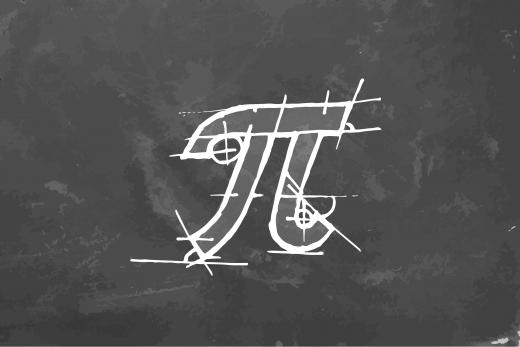# What is Pi?

Pi (π), is the 16th letter of the Greek alphabet, and it is used to represent the most widely known mathematical constant. By definition, Pi is the ratio of the circumference of a circle to its diameter. In other words, Pi equals the circumference divided by the diameter (π = c/d). Conversely, the circumference is equal to Pi times the diameter (c = πd). No matter how large or small a circle is, Pi will always work out to be the same number. AMAZING!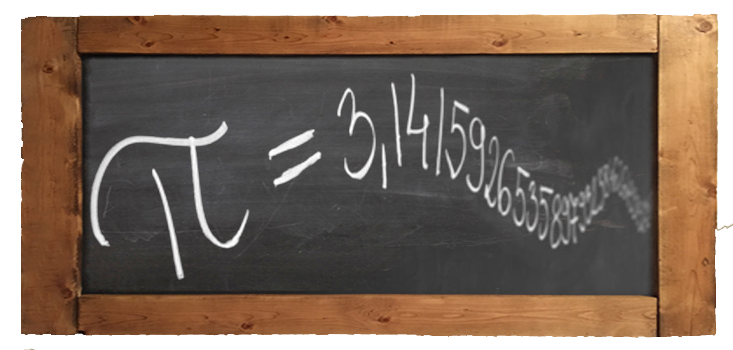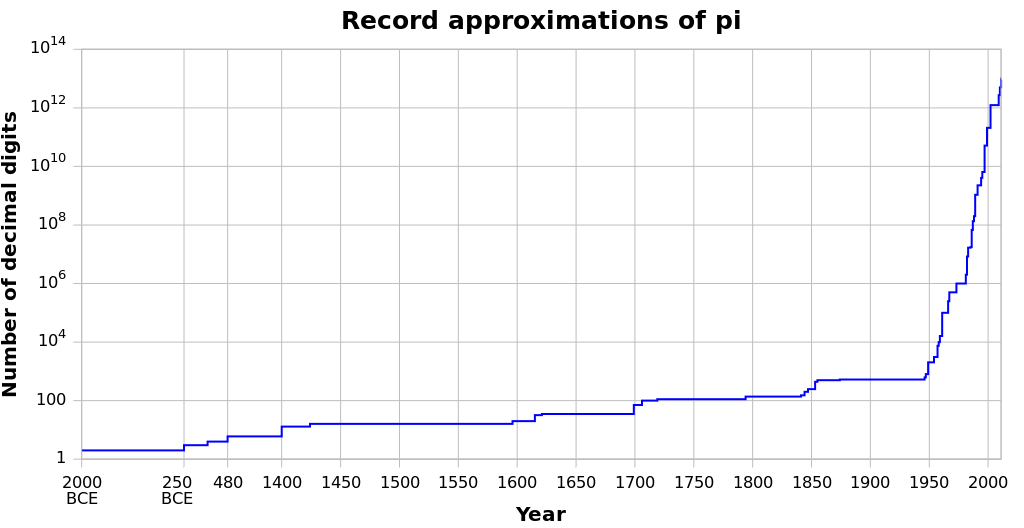# π approximation over time

This graph depicts the historical evolution of record precision numerical approximations of pi, measured in decimal places.

# CS670 π Project

In celebration of Pi, Dr. Geoffrey Exoo's Concurrent Programming Class endeavored to calculate 1 millinon digits of the this esteemed constant. Several strategies were used, including algorithms using Machin-like formulas and the Chudnovsky algorithm.#### Ali N Salman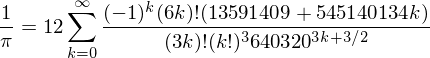(Chudnovsky algorithm)#### Abhijit Chintagunta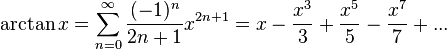(Machin-like formula)#### Sharath Raini(Machin-like formula)#### Siva Dharman Naidu(Machin-like formula)

# π Fun Facts

How many ISU phone numbers (812-237-xxxx) can be found within the first 200 million digits of π?
Total 223 passable phone number were found, the first phone number (812)-237-8128 occurs as first number in 32645 digit.

How many would expect ISU zipcode (47809) to occur within first 200 million digits of π?
47809 occurs 2015 times with in first 200 million of π number.